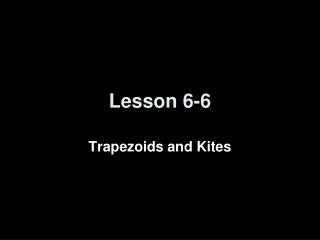DownloadDownload PresentationLesson 6-6

# Lesson 6-6

Télécharger la présentation## Lesson 6-6

- - - - - - - - - - - - - - - - - - - - - - - - - - - E N D - - - - - - - - - - - - - - - - - - - - - - - - - - -
##### Presentation Transcript

1. Lesson 6-6 Trapezoids and Kites

2. 5-Minute Check on Lesson 6-5 L • LMNO is a rhombus. • Find x • Find y • QRST is a square. • Find n if mTQR = 8n + 8. • Find w if QR = 5w + 4 and RS = 2(4w – 7). • Find QU if QS = 16t – 14 and QU = 6t + 11. • 6. What property applies to a square, but not to a rhombus? (8y – 6)° (3x + 12)° 7 M O P 12 (5x – 2)° N Q R 10.25 U 6 T S 65 Standardized Test Practice: A C Opposite sides are congruent Diagonals bisect each other D B D Opposite angles are congruent All angles are right angles Click the mouse button or press the Space Bar to display the answers.

3. Objectives • Recognize and apply the properties of trapezoids • Solve problems involving medians of trapezoids • Apply properties of kites

4. Vocabulary • Trapezoid – a quadrilateral with only one pair of parallel sides • Isosceles Trapezoid – a trapezoid with both legs (non parallel sides) congruent • Median – a segment that joins the midpoints of the legs of a trapezoid

5. Polygon Hierarchy Polygons Quadrilaterals Parallelograms Kites Trapezoids IsoscelesTrapezoids Rectangles Rhombi Squares

6. Trapezoids Trapezoid CharacteristicsBases Parallel Legs are not Parallel Leg angles are supplementary (mA + mC = 180, mB + mD = 180) Median is parallel to basesMedian = ½ (base + base)½(AB + CD) base A B legmidpoint legmidpoint median C D base A B Isosceles Trapezoid CharacteristicsLegs are congruent (AC  BD) Base angle pairs congruent (A  B, C  D) Diagonals are congruent (AD  BC) M C D

7. Kites Characteristics Two pairs of consecutive sides congruent AB  AD and CB  CD Diagonals are perpendicular AC  BD Diagonals are angle bisectors BAC  DAC and ABD  CBD ADB  BDC and BCA  DCA Diagonal from noncongruent angles bisects other diagonal diagonal BD is cut in half Only one pair of opposite angles are congruent (the pair of angles formed by the non-congruent sides)ABC  ADC A D B C

8. Example 1a A. If WXYZ is a kite, find mXYZ. Since a kite only has one pair of congruent angles, which are between the two non-congruent sides, WXY  WZY. So, WZY = 121. mW + mX + mY + mZ = 360 Polygon Interior Angles Sum Theorem 73 + 121 + mY + 121 = 360 Substitution mY = 45 Simplify. Answer: mXYZ = 45

9. Example 1b B. If WXYZ is a kite, find NP. Since the diagonals of a kite are perpendicular, they divide MNPQ into four right triangles. Use the Pythagorean Theorem to find MN, the length of the hypotenuse of right ΔMNR. NR2 + MR2 = MN2 Pythagorean Theorem (6)2 + (8)2 = MN2 Substitution 36 + 64 = MN2 Simplify. 100 = MN2 Add. 10 = MN Take the square root of each side. Answer: Since MN  NP, MN = NP. By substitution, NP = 10

10. Example 2 The top of this work station appears to be two adjacent trapezoids. Determine if they are isosceles trapezoids. Each pair of base angles is congruent, so the legs are the same length. Answer: Both trapezoids are isosceles.

11. Example 3 The sides of a picture frame appear to be two adjacent trapezoids. Determine if they are isosceles trapezoids. Answer: yes

12. DEFG is an isosceles trapezoid with median Find DG if and Answer: Example 4a Theorem 8.20 Substitution Multiply each side by 2. Subtract 20 from each side.

13. DEFG is an isosceles trapezoid with median Find , and if and Example 4b Since EF // DG, 1 and 3 are supplementary Because this is an isosceles trapezoid, 1  2 and 3  4 Substitution Combine like terms. Divide each side by 9. Answer: If x = 20, then m1 = 65° and 3 = 115°. Because 1  2 and 3  4, 2 = 65° and 4 = 115°

14. WXYZ is an isosceles trapezoid with median a. Answer: b. Answer: Because Example 5

15. Quadrilateral Characteristics Summary Convex Quadrilaterals 4 sided polygon 4 interior angles sum to 360 4 exterior angles sum to 360 Parallelograms Trapezoids Bases Parallel Legs are not Parallel Leg angles are supplementary Median is parallel to basesMedian = ½ (base + base) Opposite sides parallel and congruent Opposite angles congruent Consecutive angles supplementary Diagonals bisect each other Kites 2 congruent sides (consecutive) Diagonals perpendicular Diagonals bisect opposite angles One diagonal bisected One pair of opposite angle congruent Rectangles Rhombi IsoscelesTrapezoids Angles all 90° Diagonals congruent All sides congruent Diagonals perpendicular Diagonals bisect opposite angles Legs are congruent Base angle pairs congruent Diagonals are congruent Squares Diagonals divide into 4 congruent triangles

16. Summary & Homework • Summary: • In an isosceles trapezoid, both pairs of base angles are congruent and the diagonals are congruent. • The median of a trapezoid is parallel to the bases and its measure is one-half the sum of the measures of the bases • Homework: • pg 440-42; 1, 6, 7, 16-21, 41